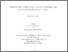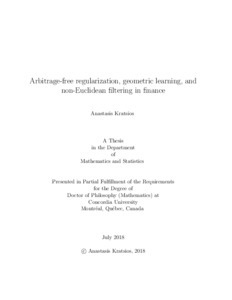Title:

# Arbitrage-free regularization, geometric learning, and non-Euclidean filtering in finance

Kratsios, Anastasis (2018) Arbitrage-free regularization, geometric learning, and non-Euclidean filtering in finance. PhD thesis, Concordia University.Preview
Text (application/pdf)
Kratsios_PhD_F2018.pdf - Accepted Version
2MB

## Abstract

This thesis brings together elements of differential geometry, machine learning, and pathwise stochastic analysis to answer problems in mathematical finance. The overarching theme is the development of new stochastic machine learning algorithms which incorporate arbitrage-free and geometric features into their estimation procedures in order to give more accurate forecasts and preserve the geometric and financial structure in the data.

This thesis is divided into three part. The first part introduces the non-Euclidean upgrading (NEU) meta-algorithm which builds the universal reconfiguration and universal approximation properties into any objective learning algorithm. These properties state that a procedure can reproduce any dataset exactly and approximate any function to arbitrary precision, respectively. This is done through an unsupervised learning procedure which identifies a geometry optimizing the relationship between a dataset and the objective learning algorithm used to explain it. The effectiveness of this procedure is supported both theoretically and numerically. The numerical implementations find that NEU-ordinary least squares outperforms leading regularized regression algorithms and that NEU-PCA explains more variance with one NEU-principal component than PCA does with four classical principal components.

The second part of the thesis introduces a computationally efficient characterization of intrinsic conditional expectation for Cartan-Hadamard manifolds. This alternative characterization provides an explicit way of computing non-Euclidean conditional expectation by using geometric transformations of specific Euclidean conditional expectations. This reduces many non-convex intrinsic estimation problems to transformations of well studied Euclidean conditional expectations. As a consequence, computationally tractable non-Euclidean filtering equations are derived and used to successfully forecast efficient portfolios by exploiting their geometry.

The third and final part of this thesis introduces a flexible modeling framework and a stochastic learning methodology for incorporating arbitrage-free features into many asset price models. The procedure works by minimally deforming the structure of a model until the objective measure acts as a martingale measure for that model. Reformulations of classical no-arbitrage results such as NFLVR, the minimal martingale measure, and the arbitrage-free Nelson-Siegel correction of the Nelson-Siegel model are all derived as solutions to specific arbitrage-free regularization problems. The flexibility and generality of this framework allows classical no-arbitrage pricing theory to be extended to models that admit arbitrage opportunities but are deformable into arbitrage-free models. Numerical implications are investigated in each of the three parts making up this thesis.

Divisions: Concordia University > Faculty of Arts and Science > Mathematics and Statistics Thesis (PhD) Kratsios, Anastasis Concordia University Ph. D. Mathematics 17 July 2018 Hyndman, Cody and Stancu, Alina machine learning, mathematical finance, computational finance, arbitrage theory, geometric learning, filtering 984318 ANASTASIS KRATSIOS 31 Oct 2018 17:37 31 Oct 2018 17:37
All items in Spectrum are protected by copyright, with all rights reserved. The use of items is governed by Spectrum's terms of access.

Repository Staff Only: item control pageResearch related to the current document (at the CORE website)
Back to top